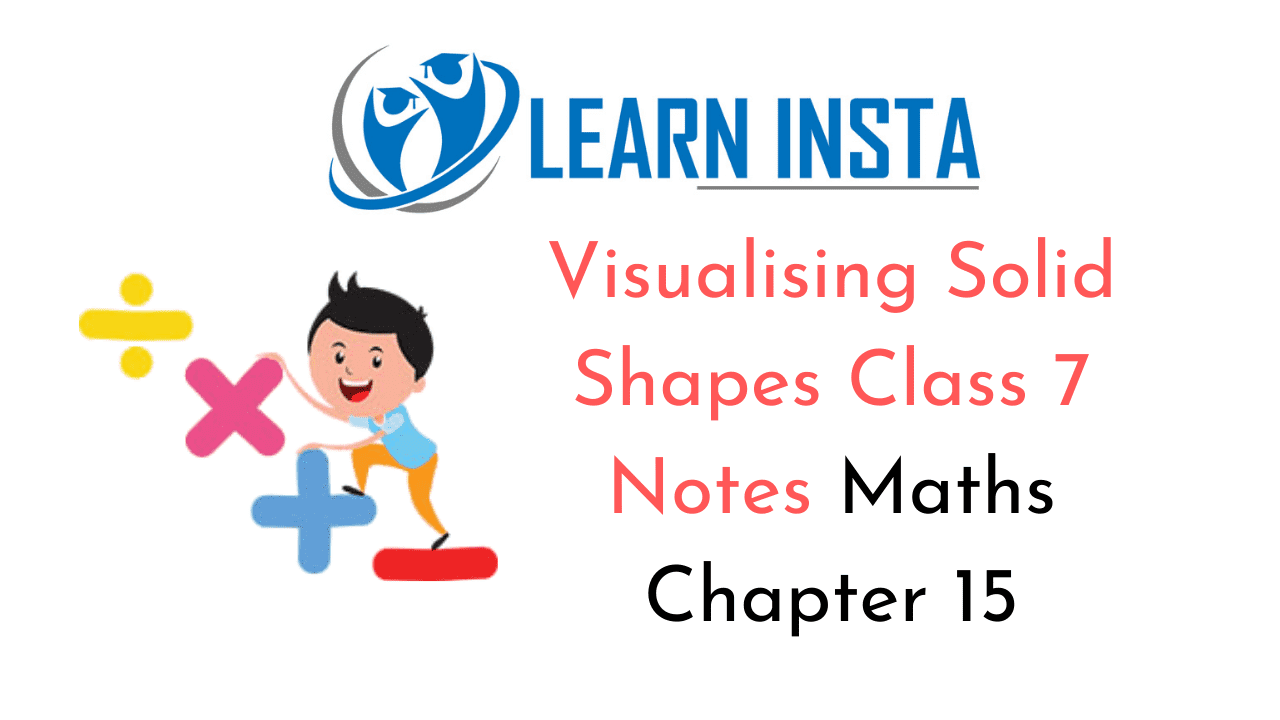On this page, you will find Visualising Solid Shapes Class 7 Notes Maths Chapter 15 Pdf free download. CBSE NCERT Class 7 Maths Notes Chapter 15 Visualising Solid Shapes will seemingly help them to revise the important concepts in less time.

## CBSE Class 7 Maths Chapter 15 Notes Visualising Solid Shapes

### Visualising Solid Shapes Class 7 Notes Conceptual Facts

Solid Shapes:
1. Cuboid: Cuboid has length, breadth and height.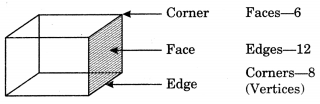2. Cube: Cube has all sides equal.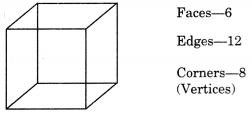3. Prism:
(i) Triangular prism: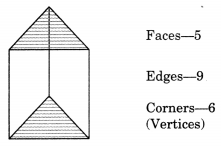(ii) Rectangular prism:4. Pyramid:
(i) Triangular pyramid(ii) Rectangular pyramid: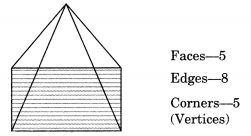5. Tetrahedron: A triangle pyramid whose all the face are equilateral triangles of same size.

6. Cylinder:7. Cone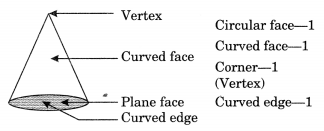8. Sphere:Euler’s Formula:
V + F – E = 2
Net of 3-D shapes: Net is an arrangement of figures connected with their edges in the same plane.

(i) Net of cube: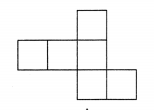(ii) Net of  Cylinder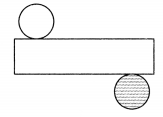(iii) Net of pyramid:(iv) Net of Cone